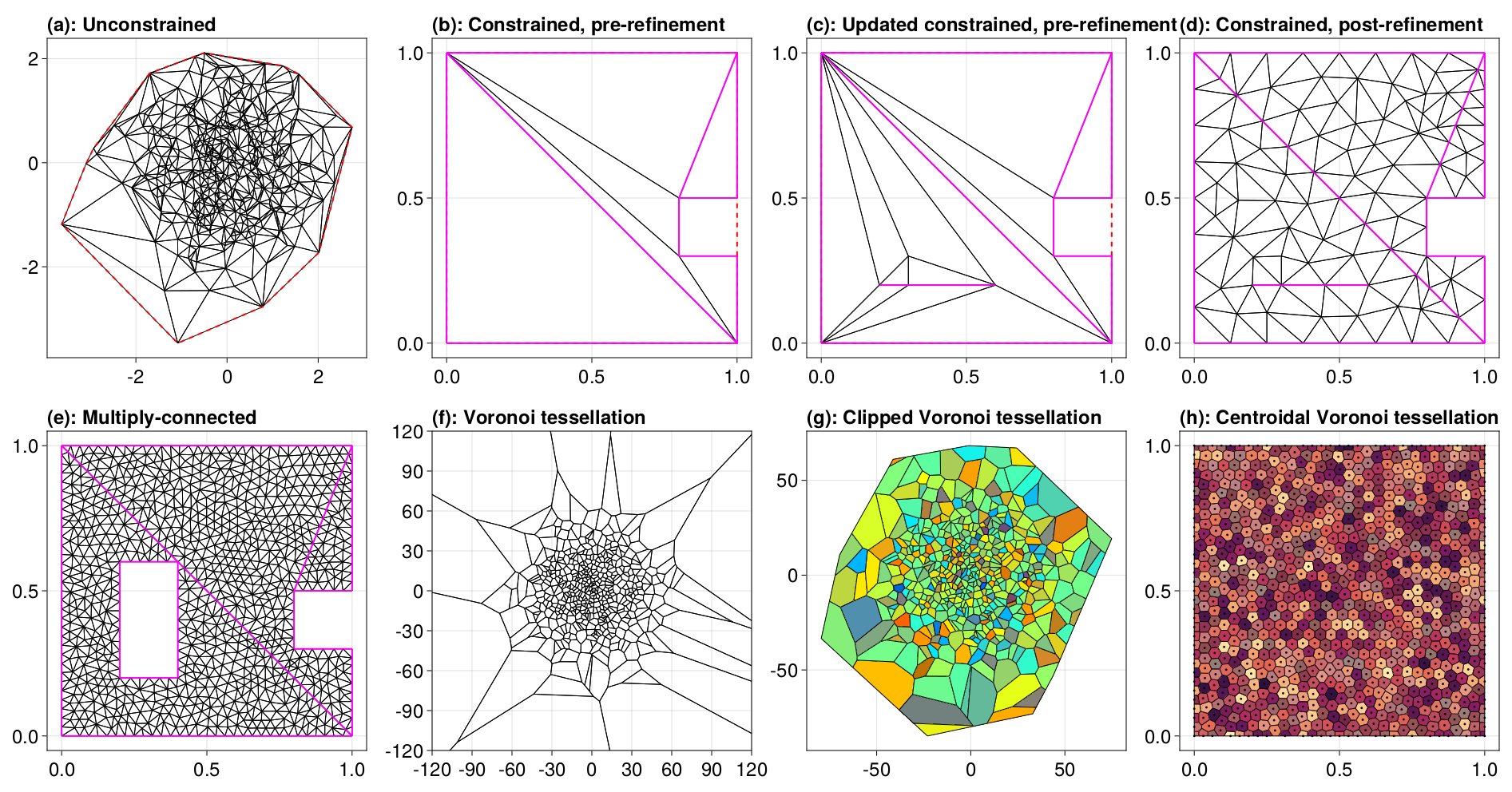## DelaunayTriangulation.jl

Delaunay triangulations and Voronoi tessellations of planar point sets.
Author DanielVandH
Popularity
25 Stars
Updated Last
4 Months Ago
Started In
September 2022

# DelaunayTriangulation

This is a package for constructing Delaunay triangulations and Voronoi tessellations of planar point sets. Supports unconstrained and constrained triangulations, mesh refinement, Voronoi tessellations, and clipped and centroidal Voronoi tessellations. All geometric predicates are computed via ExactPredicates.jl. Many features are available, some of these being:

Much of the work in this package is derived from the book Delaunay Mesh Generation by Cheng, Dey, and Shewchuk (2013). Feel free to use the issues tab for any suggestions, feedback, or if you have any questions about using the package, internals, etc.

## Quick Example

See the docs for plenty of examples. For now, here are some small examples.

using DelaunayTriangulation, CairoMakie, StableRNGs

fig = Figure(fontsize=24)

## Unconstrained example: Just some random points
rng = StableRNG(2)
pts = randn(rng, 2, 500)
tri = triangulate(pts; rng)
ax = Axis(fig[1, 1], title = "(a): Unconstrained", titlealign = :left, width=400,height=400)
triplot!(ax, tri)

## Constrained example: Generate some points, convert to indices, triangulate
points = [(0.0, 0.0), (1.0, 0.0), (1.0, 0.3), (0.8, 0.3),
(0.8, 0.5), (1.0, 0.5), (1.0, 1.0), (0.0, 1.0), (0.0, 0.0)]
boundary_nodes, points = convert_boundary_points_to_indices(points)
edges = Set(((2, 8), (5, 7)))
tri = triangulate(points; boundary_nodes, edges, rng)
ax = Axis(fig[1, 2], title = "(b): Constrained, pre-refinement", titlealign = :left, width=400,height=400)
triplot!(ax, tri)

## Dynamic updating
n = num_points(tri)
delete_point!(tri, n+2; rng)
ax = Axis(fig[1, 3], title = "(c): Updated constrained, pre-refinement", titlealign = :left, width=400,height=400)
triplot!(ax, tri)

## Refinement example
A = get_total_area(tri)
refine!(tri; max_area = 1e-2A, min_angle = 28.7, rng)
ax = Axis(fig[1, 4], title = "(d): Constrained, post-refinement", titlealign = :left, width=400,height=400)
triplot!(ax, tri, show_convex_hull = false)

## Constrained example with holes
outer_boundary = [[(0.0, 0.0), (1.0, 0.0), (1.0, 0.3), (0.8, 0.3),
(0.8, 0.5), (1.0, 0.5), (1.0, 1.0), (0.0, 1.0), (0.0, 0.0)]]
inner_boundary = [[(0.2, 0.2), (0.2, 0.6), (0.4, 0.6), (0.4, 0.2), (0.2, 0.2)]]
boundary_nodes, points = convert_boundary_points_to_indices([outer_boundary, inner_boundary])
edges = Set(((2, 8), (5, 7)))
tri = triangulate(points; boundary_nodes, edges, rng)
A = get_total_area(tri)
refine!(tri; max_area = 1e-3A, min_angle = 31.5, rng)
ax = Axis(fig[2, 1], title = "(e): Multiply-connected", titlealign = :left, width=400,height=400)
triplot!(ax, tri, show_convex_hull = false)

## Voronoi tessellation: Make tessellations from their dual triangulation
pts = 25randn(rng, 2, 500)
tri = triangulate(pts; rng)
vorn = voronoi(tri)
ax = Axis(fig[2, 2], title = "(f): Voronoi tessellation", titlealign = :left, width=400,height=400)
voronoiplot!(ax, vorn, show_generators = false)
xlims!(ax, -120, 120); ylims!(ax, -120, 120)

## Clipped Voronoi tessellation
vorn = voronoi(tri, true)
colors = get_polygon_colors(vorn, cmap)
ax = Axis(fig[2, 3], title = "(g): Clipped Voronoi tessellation", titlealign = :left, width=400,height=400)
voronoiplot!(ax, vorn, show_generators = false, polygon_color = colors)

## Centroidal Voronoi tessellation (CVT)
points = [(0.0,0.0),(1.0,0.0),(1.0,1.0),(0.0,1.0)]
tri = triangulate(points; boundary_nodes = [1,2,3,4,1], rng)
refine!(tri; max_area=1e-3, min_angle = 29.871, rng)
vorn = voronoi(tri)
smooth_vorn = centroidal_smooth(vorn; maxiters = 2500)
colors = get_polygon_colors(smooth_vorn, cmap)
ax = Axis(fig[2, 4], title = "(h): Centroidal Voronoi tessellation", titlealign = :left, width=400,height=400)
voronoiplot!(ax, smooth_vorn, show_generators = true, markersize=4, polygon_color = colors)

resize_to_layout!(fig)
fig## Animations

Just as a nice demonstration of the incremental behaviour of the algorithms in this package, here's an example of how we build a triangulation of the Julia logo.

animation.mp4

Here is also a nice animation showing the computation of a centroidal Voronoi tessellation.

lloyd_animation.mp4

## Similar Packages

This is not the only Delaunay triangulation package available. Some others are:

• VoronoiDelaunay.jl: A pure Julia library that constructs planar triangulations and tessellations like in this package, although no support for constrained triangulations / mesh refinement or clipped / centroid tessellations. Restricts points to $[1, 2] \times [1, 2]$.
• VoronoiCells.jl: A pure Julia library that extends VoronoiDelaunay.jl. This package provides useful tools for constructing and working with Voronoi tessellations. Supports clipping Voronoi cells to a specified rectangle. Like VoronoiDelaunay.jl, restricts points to $[1, 2] \times [1, 2]$.
• Delaunay.jl: Wraps Python's main Delaunay triangulation library, scipy.spatial.Delaunay, for computing Delaunay triangulations in $\mathbb R^N$. I don't believe constrained triangulations or mesh refinement is available here.
• MiniQhull.jl: Wraps Qhull for computing unconstrained Delaunay triangulations in $\mathbb R^N$. No support is provided for mesh refinement.
• DirectQhull.jl: Similar to MiniQhull.jl, although also provides support for convex hulls and Voronoi tessellations from Qhull.
• Delaunator.jl: A pure Julia library modelled after the JavaScript Delaunator library. This package can construct unconstrained triangulations of planar point sets. No support is available for constrained triangulations or mesh refinement, although support exists for computing the dual Voronoi tessellation. Centroidal tessellations are not implemented, although the Voronoi cells can be clipped to a bounding box.
• TriangleMesh.jl, Triangulate.jl, Triangle.jl: Interfaces to Shewchuk's Triangle library.
• TetGen.jl: This is for Delaunay tetrahedralisation, wrapping TetGen.

### Required Packages

View all packages